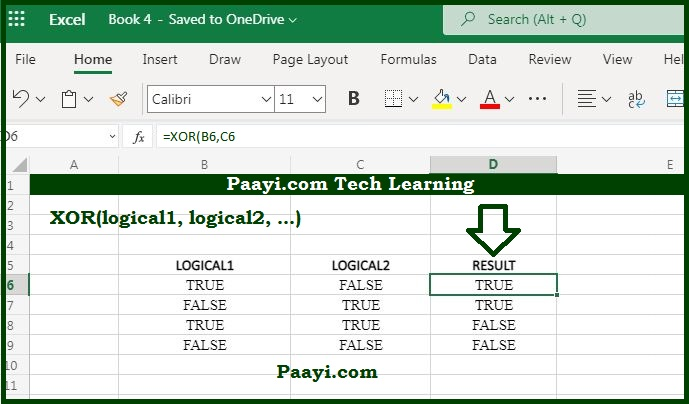# Learn How to Use Microsoft Excel XOR Function

Written by | 0 Comments | 726 Views

In this article, you will learn how to use the Microsoft Excel XOR function and its prime function in Microsoft Excel. You will also get to know the MS Excel XOR function return value and syntax with the help of some examples.

## The Microsoft Excel XOR Function

The Microsoft Excel XOR function is used to perform the exclusive OR function. So, it means the XOR function performs that is known as "exclusive OR." It is used with two logical statements; the XOR function returns TRUE if any one of them is TRUE and returns FALSE if both the statements are true. If both the statements are FALSE, the XOR function returns FALSE.

### XOR Function Return Value

TRUE or FALSE

### XOR Function Syntax

=XOR(logical1,[logical2],...)

Where:

• logical1: It can be an expression, constant, or reference that evaluates TRUE or FALSE.
• logical2: This is optional, and it can also be an expression, constant, and statement that will determine TRUE or FALSE.

## How to Use Microsoft Excel XOR Function?The operation performed by the XOR function is known as "exclusive OR," which is the opposite of "inclusive OR" performed by the OR function. The OR returns TRUE if any of the logic is TRUE, while the XOR function returns TRUE in specific cases.

In the simplest example using the two statements, the XOR will return TRUE if any one of the logical is TRUE, and not when both the logical is TRUE. Basically, the exclusive OR is more used in programming. In simple terms, when you say, "I am planning to visit Shimla or Goa this summer," that means no one is stopping you from visiting both, but you are only planning for the one.

### XOR Function With More than Two Logical

When we use more than two logical, the XOR function will return TRUE only when the number of TRUE logical is odd, as we have shown in the examples below: